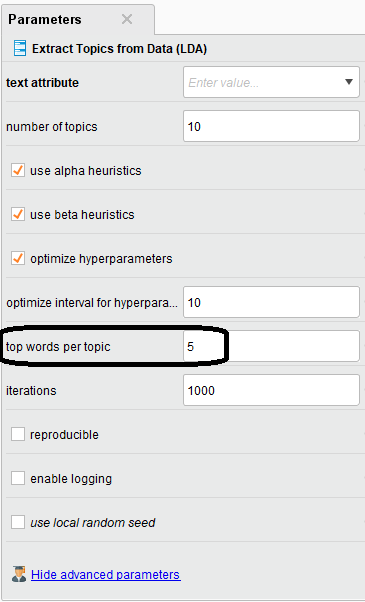# How can I find what words are in each topic after applying LDA on text ?

Member Posts: 2Newbie
edited December 2018 in Help

# LDA Model

```LDA Model with 20 topics
alphaSum = 2.0285924067154273
beta = 0.019139372553800438
Topic 0	tokens=345657.0000	document_entropy=8.0896	word-length=7.4000	coherence=-10.0817	uniform_dist=3.8276	corpus_dist=1.2224	eff_num_words=739.9272	token-doc-diff=0.0178	rank_1_docs=0.1799	allocation_ratio=0.0869	allocation_count=0.1734	exclusivity=0.2313
health	word-length=6.0000	coherence=0.0000	uniform_dist=0.0898	corpus_dist=0.0385	token-doc-diff=0.0130	exclusivity=0.8054
companies	word-length=9.0000	coherence=-1.1568	uniform_dist=0.0523	corpus_dist=0.0069	token-doc-diff=0.0000	exclusivity=0.1182
startups	word-length=8.0000	coherence=-0.9839	uniform_dist=0.0466	corpus_dist=0.0054	token-doc-diff=0.0023	exclusivity=0.1031
company	word-length=7.0000	coherence=-1.1837	uniform_dist=0.0445	corpus_dist=-0.0013	token-doc-diff=0.0014	exclusivity=0.0417
startup	word-length=7.0000	coherence=-1.0639	uniform_dist=0.0406	corpus_dist=0.0039	token-doc-diff=0.0011	exclusivity=0.0882
Topic 1	tokens=852784.0000	document_entropy=9.4027	word-length=5.6000	coherence=-9.7164	uniform_dist=4.4613	corpus_dist=0.8509	eff_num_words=302.2245	token-doc-diff=0.0012	rank_1_docs=0.1414	allocation_ratio=0.0766	allocation_count=0.1608	exclusivity=0.2635
apps	word-length=4.0000	coherence=0.0000	uniform_dist=0.2079	corpus_dist=0.0497	token-doc-diff=0.0002	exclusivity=0.3843
users	word-length=5.0000	coherence=-0.5399	uniform_dist=0.1801	corpus_dist=0.0321	token-doc-diff=0.0000	exclusivity=0.2216
mobile	word-length=6.0000	coherence=-0.6889	uniform_dist=0.1485	corpus_dist=0.0255	token-doc-diff=0.0000	exclusivity=0.1904
google	word-length=6.0000	coherence=-1.3039	uniform_dist=0.0879	corpus_dist=0.0172	token-doc-diff=0.0002	exclusivity=0.2262
android	word-length=7.0000	coherence=-1.1189	uniform_dist=0.0648	corpus_dist=0.0148	token-doc-diff=0.0008	exclusivity=0.2949
Topic 2	tokens=726725.0000	document_entropy=9.5265	word-length=7.0000	coherence=-7.0986	uniform_dist=4.7579	corpus_dist=1.1700	eff_num_words=202.4635	token-doc-diff=0.0005	rank_1_docs=0.0950	allocation_ratio=0.0282	allocation_count=0.1139	exclusivity=0.4547
million	word-length=7.0000	coherence=0.0000	uniform_dist=0.2162	corpus_dist=0.0471	token-doc-diff=0.0002	exclusivity=0.2991
company	word-length=7.0000	coherence=-0.5201	uniform_dist=0.1916	corpus_dist=0.0268	token-doc-diff=0.0001	exclusivity=0.1506
funding	word-length=7.0000	coherence=-0.5500	uniform_dist=0.1620	corpus_dist=0.0522	token-doc-diff=0.0001	exclusivity=0.7814
startup	word-length=7.0000	coherence=-0.7616	uniform_dist=0.1340	corpus_dist=0.0297	token-doc-diff=0.0001	exclusivity=0.2514
capital	word-length=7.0000	coherence=-0.8565	uniform_dist=0.1161	corpus_dist=0.03

```
Tagged:

• Moderator, RapidMiner Certified Analyst, Member Posts: 1,195Unicorn
Hi @Sanju

Have you try to modify the "top words per topics" parameter of the operator ?Hope it helps,

Regards,

Lionel
• Member Posts: 2Newbie
edited December 2018
Hi @Lionel
I already try this way, but I want to see all words in each topic